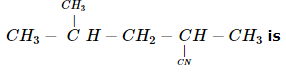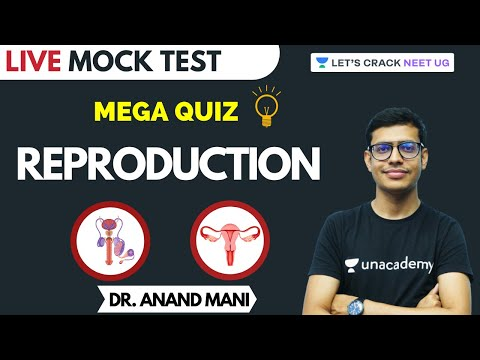Courses

# SRMJEEE Chemistry Mock Test - 4

## 35 Questions MCQ Test SRMJEEE Subject Wise & Full Length Mock Tests | SRMJEEE Chemistry Mock Test - 4

Description
This mock test of SRMJEEE Chemistry Mock Test - 4 for JEE helps you for every JEE entrance exam. This contains 35 Multiple Choice Questions for JEE SRMJEEE Chemistry Mock Test - 4 (mcq) to study with solutions a complete question bank. The solved questions answers in this SRMJEEE Chemistry Mock Test - 4 quiz give you a good mix of easy questions and tough questions. JEE students definitely take this SRMJEEE Chemistry Mock Test - 4 exercise for a better result in the exam. You can find other SRMJEEE Chemistry Mock Test - 4 extra questions, long questions & short questions for JEE on EduRev as well by searching above.
QUESTION: 1

Solution:
QUESTION: 2

Solution:
QUESTION: 3

### Reaction of alkyl halide with alcoholic KOH is called

Solution:
QUESTION: 4

The reagent which forms crystalline osazone derivative when reacted with glucose is

Solution:
QUESTION: 5

Which one of the following molecules has the highest dipole moment ?

Solution:
QUESTION: 6

When HNO₃ is dropped into the palm and washed with water, it turns yellow. It shows the presence of

Solution:
QUESTION: 7

The compound/ion which is used in the nitration of benzene is

Solution:
QUESTION: 8

The total number of possible values of magnetic quantum number for the value of l = 3 is

Solution:
QUESTION: 9

How many moles of Helium gas occupy 22.4 L at 0°C at 1 atm. pressure?

Solution:
QUESTION: 10

Carboxylic acids are more acidic than phenol and alcohol because of

Solution:
QUESTION: 11

Number of sigma bonds in P₄O₁₀ is

Solution:
QUESTION: 12

The rate constant of a reaction depends on

Solution:
QUESTION: 13

A chemical reaction is catalyzed by a catalyst X. Hence X

Solution:
QUESTION: 14

One gram sample of NH4NO3 is decomposed in a bomb-calorimeter. Temperature of the calorimeter increases by 6.12 K. Heat capacity of the system is 1.23 KJ-g-1 - deg-1. What is the molar heat of decomposition for NH4NO3 is

Solution:

ΔU = - 6.12 x 1.23 x GMM (NH4NO3)
= - 6.12 x 1.23 x 80 = - 602.208 kJ mol-1

QUESTION: 15

In the reaction CH2COOHaq + OH(aq) → CH3COO- + H2O(l) ; ΔH = -55.2kJ mol-1. What is the heat of dissociation (in kJ mol-1) of acetic acid?

Solution:

ΔH = -55.2 KJ.mol-1
-[H+ (aq) + OH- (aq) → H2O(l) ΔH = -57.3 KJ .mol-1]]

CH2COOHaq → CH3COO- (aq) + H+ (aq) ΔH = ?
CH2COOH(aq) + OH- (aq) → CH3COO- (aq) + H2O(l) ΔH = -55.2 KJ .mol-1
-H+ + OH- (aq) → - H2O(l) ΔH = + 57.3KJ .mol-1
CH3COOH(aq) → CH3COO- (aq) + H+(aq) ΔH = 2.1 KJmol-1

QUESTION: 16

One of the most widely used drug in medicine iodex in

Solution:
QUESTION: 17

IUPAC name ofSolution:
QUESTION: 18

Conductivity (Unit : siemen's S) is directly proportionally to the area of the vessel and the concentration of the solution in it and is inversely proportional to the length of vessel, then the unit of constant of proportionality is

Solution:
QUESTION: 19

Which of the following metal requires radiation of highest frequency to cause emission of electrons?

Solution:

As the alkali metals have minimum ionisation potential in the periodic table, secondly ionisation potential decreases on moving top to bottom in periodic table, thus Mg has highest ionisation enthalpy among the given elements, hence it requires radiation of highest frequency to cause emission of electrons.

QUESTION: 20

A real gas most closely approaches the behaviour of an ideal gas at

Solution:
QUESTION: 21

Benzene reacts with n-propyl chloride in the presence of anhydrous AlCl₃ to give predominantly

Solution:
QUESTION: 22

Hydrogen ion concentration of 0.2 M CH₃COOH, which is 40% dissociated, is

Solution:
QUESTION: 23

In which of the following separation method only one enantiomer is recovered from the racemic mixture?

Solution:
QUESTION: 24

Bismuth is the end product of radioactive disintegration series known as

Solution:
QUESTION: 25

Which of the following is a strongest base?

Solution:
QUESTION: 26

The reduction of benzoyl chloride with H₂/Pd-BaSO₄ produces

Solution:
QUESTION: 27

Which of the following is an example of natural polymer?

Solution:
QUESTION: 28

Molecular mass of a volatile substance may be obtained by

Solution:
QUESTION: 29

Which is the strongest reducing agent ?

Solution:
QUESTION: 30

Which of the following is anti-ferromagnetism?

Solution:
QUESTION: 31

30 ml of solution is neutralised by 15 ml of 0.2 N base. The strength of the acid solution is

Solution:
QUESTION: 32

Which comparison is not correct as indicated?

Solution:
QUESTION: 33

The vapour pressure of water at 300 K in a closed cotainer is 0.4 atm. If the volume of container is doubled. The vapour pressure at 300 K will be

Solution:
QUESTION: 34

In which one of the following reactions does SO3 acts as an oxidising agent reaction with

Solution:
QUESTION: 35

Which of the following is used for the destruction of colloids?

Solution:

Track your progress, build streaks, highlight & save important lessons and more!

### Similar Content### Related tests